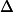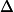# A 270-kg load is lifted.....

## Homework Statement

A 270-kg load is lifted 21.0 m vertically with an acceleration a=0.150 g by a single cable.

A)Determine the tension in the cable.
B)Determine the net work done on the load.
C)Determine the work done by gravity on the load.
D)Determine the final speed of the load assuming it started from rest.

## The Attempt at a Solution

i was able to get both A and B, for A my answer was....
FT =
3040 N

and for B the answer i got was
Wnet =
8330 J

RUber
Homework Helper
For part D, the speed is based on basic mechanics and your acceleration.
## v_f = \int_{t_0}^{t_f} a dt ##
Assuming t_0 = 0 and v_0 = 0, you will get an answer for v_f based on total time.
To find total time, you integrate once more, and solve for how long it takes to go 21m.

For part C, I am not too sure, but I would treat gravity like any other force and plug it into your equation for work.
One I found on the internet is W= -mg(h). The negative sign picked up since gravity is working against the distance of travel in this case.

For part D, the speed is based on basic mechanics and your acceleration.
## v_f = \int_{t_0}^{t_f} a dt ##
Assuming t_0 = 0 and v_0 = 0, you will get an answer for v_f based on total time.
To find total time, you integrate once more, and solve for how long it takes to go 21m.

For part C, I am not too sure, but I would treat gravity like any other force and plug it into your equation for work.
One I found on the internet is W= -mg(h). The negative sign picked up since gravity is working against the distance of travel in this case.

i am so sorry but i do not understand? could you elaborate?

SammyS
Staff Emeritus
Homework Helper
Gold Member

## Homework Statement

A 270-kg load is lifted 21.0 m vertically with an acceleration a=0.150 g by a single cable.

A)Determine the tension in the cable.
B)Determine the net work done on the load.
C)Determine the work done by gravity on the load.
D)Determine the final speed of the load assuming it started from rest.

## The Attempt at a Solution

i was able to get both A and B, for A my answer was....
FT =
3040 N

and for B the answer i got was
Wnet =
8330 J

How did you get the answers for A and for B ?

C is similar.

For D, use simple kinematics, or use the work-energy theorem.

RUber
Homework Helper
i am so sorry but i do not understand? could you elaborate?
I only learned calc-based physics, so I am not sure how to be more clear.
Velocity is the integral of acceleration.
For a constant acceleration, you have ##v(t) = at + v_0##
In this case, v_0 is 0 because it says the object starts at rest.

Position is the integral of velocity.
For a linear velocity, like v = at, position ##p(t) = \frac a2 t^2 +p_0##.
In this case, you want to find the time it takes for p(t) = 21, with p_0 = 0.
This will tell you how long it takes to raise the object to 21m.

Once you have the time, you plug that into your linear equation for velocity to solve the problem.